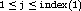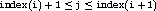## 159. General (Graph) ConstructorUp: Topology Constructors Next: Topology Inquiry Functions Previous: Cartesian Convenience Function: MPI_DIMS_CREATE

 MPI_GRAPH_CREATE(comm_old, nnodes, index, edges, reorder, comm_graph) IN comm_old input communicator (handle) IN nnodes number of nodes in graph (integer) IN index array of integers describing node degrees (see below) IN edges array of integers describing graph edges (see below) IN reorder ranking may be reordered ( true) or not ( false) (logical) OUT comm_graph communicator with graph topology added (handle)

int MPI_Graph_create(MPI_Comm comm_old, int nnodes, int *index, int *edges, int reorder, MPI_Comm *comm_graph)

MPI_GRAPH_CREATE(COMM_OLD, NNODES, INDEX, EDGES, REORDER, COMM_GRAPH, IERROR)
INTEGER COMM_OLD, NNODES, INDEX(*), EDGES(*), COMM_GRAPH, IERROR
LOGICAL REORDER
MPI::Graphcomm MPI::Intracomm::Create_graph(int nnodes, const int index[], const int edges[], bool reorder) const
MPI_GRAPH_CREATE returns a handle to a new communicator to which the graph topology information is attached. If reorder = false then the rank of each process in the new group is identical to its rank in the old group. Otherwise, the function may reorder the processes. If the size, nnodes, of the graph is smaller than the size of the group of comm, then some processes are returned MPI_COMM_NULL, in analogy to MPI_CART_CREATE and MPI_COMM_SPLIT. If the graph is empty, i.e., nnodes == 0, then MPI_COMM_NULL is returned in all processes. The call is erroneous if it specifies a graph that is larger than the group size of the input communicator.

The three parameters nnodes, index and edges define the graph structure. nnodes is the number of nodes of the graph. The nodes are numbered from 0 to nnodes-1. The i-th entry of array index stores the total number of neighbors of the first i graph nodes. The lists of neighbors of nodes 0, 1, ..., nnodes-1 are stored in consecutive locations in array edges. The array edges is a flattened representation of the edge lists. The total number of entries in index is nnodes and the total number of entries in edges is equal to the number of graph edges.

The definitions of the arguments nnodes, index, and edges are illustrated with the following simple example.

Example

Assume there are four processes 0, 1, 2, 3 with the following adjacency matrix:
 process neighbors 0 1, 3 1 0 2 3 3 0, 2

Then, the input arguments are:
 nnodes = 4 index = 2, 3, 4, 6 edges = 1, 3, 0, 3, 0, 2

Thus, in C, index is the degree of node zero, and index[i] - index[i-1] is the degree of node i, i=1, ..., nnodes-1; the list of neighbors of node zero is stored in edges[j], forand the list of neighbors of node i, i > 0, is stored in edges[j],.

In Fortran, index(1) is the degree of node zero, and index(i+1) - index(i) is the degree of node i, i=1, ..., nnodes-1; the list of neighbors of node zero is stored in edges(j), forand the list of neighbors of node i, i > 0, is stored in edges(j),.

A single process is allowed to be defined multiple times in the list of neighbors of a process (i.e., there may be multiple edges between two processes). A process is also allowed to be a neighbor to itself (i.e., a self loop in the graph). The adjacency matrix is allowed to be non-symmetric.

Performance implications of using multiple edges or a non-symmetric adjacency matrix are not defined. The definition of a node-neighbor edge does not imply a direction of the communication. ( End of advice to users.)

The following topology information is likely to be stored with a communicator:

• Type of topology (Cartesian/graph),
• For a Cartesian topology:
1. ndims (number of dimensions),
2. dims (numbers of processes per coordinate direction),
3. periods (periodicity information),
4. own_position (own position in grid, could also be computed from rank and dims)
• For a graph topology:
1. index,
2. edges,
which are the vectors defining the graph structure.

For a graph structure the number of nodes is equal to the number of processes in the group. Therefore, the number of nodes does not have to be stored explicitly. An additional zero entry at the start of array index simplifies access to the topology information. ( End of advice to implementors.)Up: Topology Constructors Next: Topology Inquiry Functions Previous: Cartesian Convenience Function: MPI_DIMS_CREATE